Fundamental Equilibrium Concepts

# 80 Shifting Equilibria: Le Châtelier’s Principle

### Learning Objectives

By the end of this section, you will be able to:

• Describe the ways in which an equilibrium system can be stressed
• Predict the response of a stressed equilibrium using Le Châtelier’s principle

A system at equilibrium is in a state of dynamic balance, with forward and reverse reactions taking place at equal rates. If an equilibrium system is subjected to a change in conditions that affects these reaction rates differently (a stress), then the rates are no longer equal and the system is not at equilibrium. The system will subsequently experience a net reaction in the direction of greater rate (a shift) that will re-establish the equilibrium. This phenomenon is summarized by Le Châtelier’s principle: if an equilibrium system is stressed, the system will experience a shift in response to the stress that re-establishes equilibrium.

Reaction rates are affected primarily by concentrations, as described by the reaction’s rate law, and temperature, as described by the Arrhenius equation. Consequently, changes in concentration and temperature are the two stresses that can shift an equilibrium.

### Effect of a Change in Concentration

If an equilibrium system is subjected to a change in the concentration of a reactant or product species, the rate of either the forward or the reverse reaction will change. As an example, consider the equilibrium reaction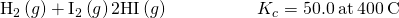The rate laws for the forward and reverse reactions are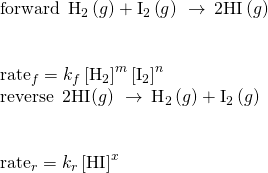When this system is at equilibrium, the forward and reverse reaction rates are equal.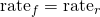If the system is stressed by adding reactant, either H2 or I2, the resulting increase in concentration causes the rate of the forward reaction to increase, exceeding that of the reverse reaction: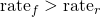The system will experience a temporary net reaction in the forward direction to re-establish equilibrium (the equilibrium will shift right). This same shift will result if some product HI is removed from the system, which decreases the rate of the reverse reaction, again resulting in the same imbalance in rates.

The same logic can be used to explain the left shift that results from either removing reactant or adding product to an equilibrium system. These stresses both result in an increased rate for the reverse reaction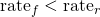and a temporary net reaction in the reverse direction to re-establish equilibrium.

As an alternative to this kinetic interpretation, the effect of changes in concentration on equilibria can be rationalized in terms of reaction quotients. When the system is at equilibrium,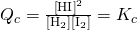If reactant is added (increasing the denominator of the reaction quotient) or product is removed (decreasing the numerator), then Qc < Kc and the equilibrium will shift right. Note that the three different ways of inducing this stress result in three different changes in the composition of the equilibrium mixture. If H2 is added, the right shift will consume I2 and produce HI as equilibrium is re-established, yielding a mixture with a greater concentrations of H2 and HI and a lesser concentration of I2 than was present before. If I2 is added, the new equilibrium mixture will have greater concentrations of I2 and HI and a lesser concentration of H2. Finally, if HI is removed, the new equilibrium mixture will have greater concentrations of H2 and I2 and a lesser concentration of HI. Despite these differences in composition, the value of the equilibrium constant will be the same after the stress as it was before (per the law of mass action). The same logic may be applied for stresses involving removing reactants or adding product, in which case Qc > Kc and the equilibrium will shift left.

For gas-phase equilibria such as this one, some additional perspectives on changing the concentrations of reactants and products are worthy of mention. The partial pressure P of an ideal gas is proportional to its molar concentration M,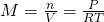and so changes in the partial pressures of any reactant or product are essentially changes in concentrations and thus yield the same effects on equilibria. Aside from adding or removing reactant or product, the pressures (concentrations) of species in a gas-phase equilibrium can also be changed by changing the volume occupied by the system. Since all species of a gas-phase equilibrium occupy the same volume, a given change in volume will cause the same change in concentration for both reactants and products. In order to discern what shift, if any, this type of stress will induce the stoichiometry of the reaction must be considered.

At equilibrium, the reaction H2(g) + I2(g) ⇌ 2HI(g) is described by the reaction quotient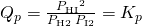If the volume occupied by an equilibrium mixture of these species is decreased by a factor of 3, the partial pressures of all three species will be increased by a factor of 3: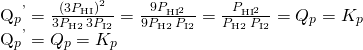And so, changing the volume of this gas-phase equilibrium mixture does not results in a shift of the equilibrium.

A similar treatment of a different system, 2NO2(g) ⇌ 2 NO(g) + O2(g), however, yields a different result: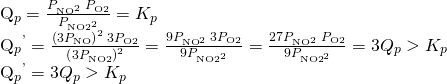In this case, the change in volume results in a reaction quotient greater than the equilibrium constant, and so the equilibrium will shift left.

These results illustrate the relationship between the stoichiometry of a gas-phase equilibrium and the effect of a volume-induced pressure (concentration) change. If the total molar amounts of reactants and products are equal, as in the first example, a change in volume does not shift the equilibrium. If the molar amounts of reactants and products are different, a change in volume will shift the equilibrium in a direction that better “accommodates” the volume change. In the second example, two moles of reactant (NO2) yield three moles of product (2NO + O2), and so decreasing the system volume causes the equilibrium to shift left since the reverse reaction produces less gas (2 mol) than the forward reaction (3 mol). Conversely, increasing the volume of this equilibrium system would result in a shift towards products.

Equilibrium and Soft Drinks

The connection between chemistry and carbonated soft drinks goes back to 1767, when Joseph Priestley (1733–1804) developed a method of infusing water with carbon dioxide to make carbonated water. Priestly’s approach involved production of carbon dioxidey reacting oil of vitriol (sulfuric acid) with chalk (calcium carbonate).

The carbon dioxide was then dissolved in water, reacting to produce hydrogen carbonate, a weak acid that subsequently ionized to yield bicarbonate and hydrogen ions: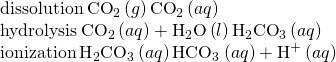These same equilibrium reactions are the basis of today’s soft-drink carbonation process. Beverages are exposed to a high pressure of gaseous carbon dioxide during the process to shift the first equilibrium above to the right, resulting in desirably high concentrations of dissolved carbon dioxide and, per similar shifts in the other two equilibria, its hydrolysis and ionization products. A bottle or can is then nearly filled with the carbonated beverage, leaving a relatively small volume of air in the container above the beverage surface (the headspace) before it is sealed. The pressure of carbon dioxide in the container headspace is very low immediately after sealing, but it rises as the dissolution equilibrium is re-established by shifting to the left. Since the volume of the beverage is significantly greater than the volume of the headspace, only a relatively small amount of dissolved carbon dioxide is lost to the headspace.

When a carbonated beverage container is opened, a hissing sound is heard as pressurized CO2 escapes from the headspace. This causes the dissolution equilibrium to shift left, resulting in a decrease in the concentration of dissolved CO2 and subsequent left-shifts of the hydrolysis and ionization equilibria. Fortunately for the consumer, the dissolution equilibrium is usually re-established slowly, and so the beverage may be enjoyed while its dissolved carbon dioxide concentration remains palatably high. Once the equilibria are re-established, the CO2(aq) concentration will be significantly lowered, and the beverage acquires a characteristic taste referred to as “flat.”

Opening a soft-drink bottle lowers the CO2 pressure above the beverage, shifting the dissolution equilibrium and releasing dissolved CO2 from the beverage. (credit: modification of work by “D Coetzee”/Flickr)### Effect of a Change in Temperature

Consistent with the law of mass action, an equilibrium stressed by a change in concentration will shift to re-establish equilibrium without any change in the value of the equilibrium constant, K. When an equilibrium shifts in response to a temperature change, however, it is re-established with a different relative composition that exhibits a different value for the equilibrium constant.

To understand this phenomenon, consider the elementary reaction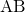Since this is an elementary reaction, the rates laws for the forward and reverse may be derived directly from the balanced equation’s stoichiometry: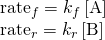When the system is at equilibrium,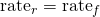Substituting the rate laws into this equality and rearranging gives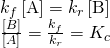The equilibrium constant is seen to be a mathematical function of the rate constants for the forward and reverse reactions. Since the rate constants vary with temperature as described by the Arrhenius equation, is stands to reason that the equilibrium constant will likewise vary with temperature (assuming the rate constants are affected to different extents by the temperature change). For more complex reactions involving multistep reaction mechanisms, a similar but more complex mathematical relation exists between the equilibrium constant and the rate constants of the steps in the mechanism. Regardless of how complex the reaction may be, the temperature-dependence of its equilibrium constant persists.

Predicting the shift an equilibrium will experience in response to a change in temperature is most conveniently accomplished by considering the enthalpy change of the reaction. For example, the decomposition of dinitrogen tetroxide is an endothermic (heat-consuming) process: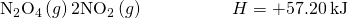For purposes of applying Le Chatelier’s principle, heat (q) may be viewed as a reactant: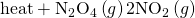Raising the temperature of the system is akin to increasing the amount of a reactant, and so the equilibrium will shift to the right. Lowering the system temperature will likewise cause the equilibrium to shift left. For exothermic processes, heat is viewed as a product of the reaction and so the opposite temperature dependence is observed.

### Effect of a Catalyst

The kinetics chapter of this text identifies a catalyst as a substance that enables a reaction to proceed via a different mechanism with an accelerated rate. The catalyzed reaction mechanism involves a lower energy transition state than the uncatalyzed reaction, resulting in a lower activation energy, Ea, and a correspondingly greater rate constant.

To discern the effect of catalysis on an equilibrium system, consider the reaction diagram for a simple one-step (elementary) reaction shown in (Figure). The lowered transition state energy of the catalyzed reaction results in lowered activation energies for both the forward and the reverse reactions. Consequently, both forward and reverse reactions are accelerated, and equilibrium is achieved more quickly but without a change in the equilibrium constant.

Reaction diagrams for an elementary process in the absence (red) and presence (blue) of a catalyst. The presence of catalyst lowers the activation energies of both the forward and reverse reactions but does not affect the value of the equilibrium constant.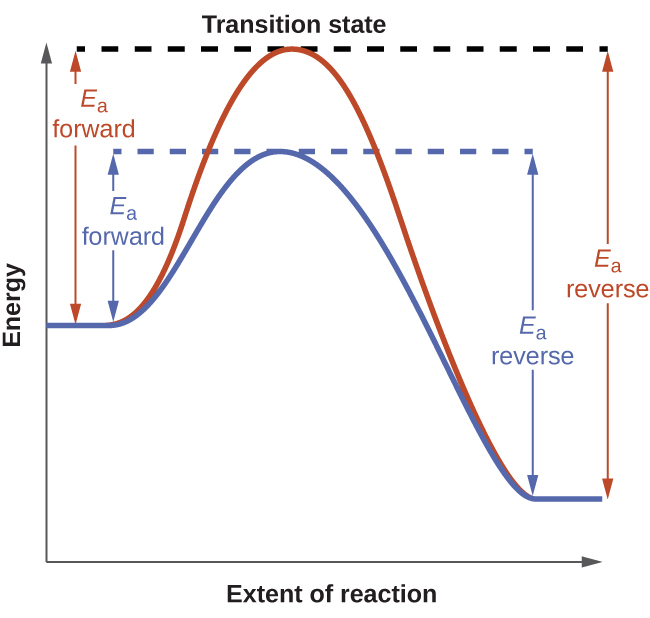An interesting case study highlighting these equilibrium concepts is the industrial production of ammonia, NH3. This substance is among the “top 10” industrial chemicals with regard to production, with roughly two billion pounds produced annually in the US. Ammonia is used as a chemical feedstock to synthesize a wide range of commercially useful compounds, including fertilizers, plastics, dyes, and explosives.

Most industrial production of ammonia uses the Haber-Bosch process based on the following equilibrium reaction: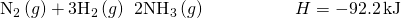The traits of this reaction present challenges to its use in an efficient industrial process. The equilibrium constant is relatively small (Kp on the order of 10−5 at 25 °C), meaning very little ammonia is present in an equilibrium mixture. Also, the rate of this reaction is relatively slow at low temperatures. To raise the yield of ammonia, the industrial process is designed to operate under conditions favoring product formation:

• High pressures (concentrations) of reactants are used, ~150−250 atm, to shift the equilibrium right, favoring product formation.
• Ammonia is continually removed (collected) from the equilibrium mixture during the process, lowering its concentration and also shifting the equilibrium right.
• Although low temperatures favor product formation for this exothermic process, the reaction rate at low temperatures is inefficiently slow. A catalyst is used to accelerate the reaction to reasonable rates at relatively moderate temperatures (400−500 °C).

A diagram illustrating a typical industrial setup for production of ammonia via the Haber-Bosch process is shown in (Figure).

The figure shows a typical industrial setup for the commercial production of ammonia by the Haber-Bosch process. The process operates under conditions that stress the chemical equilibrium to favor product formation.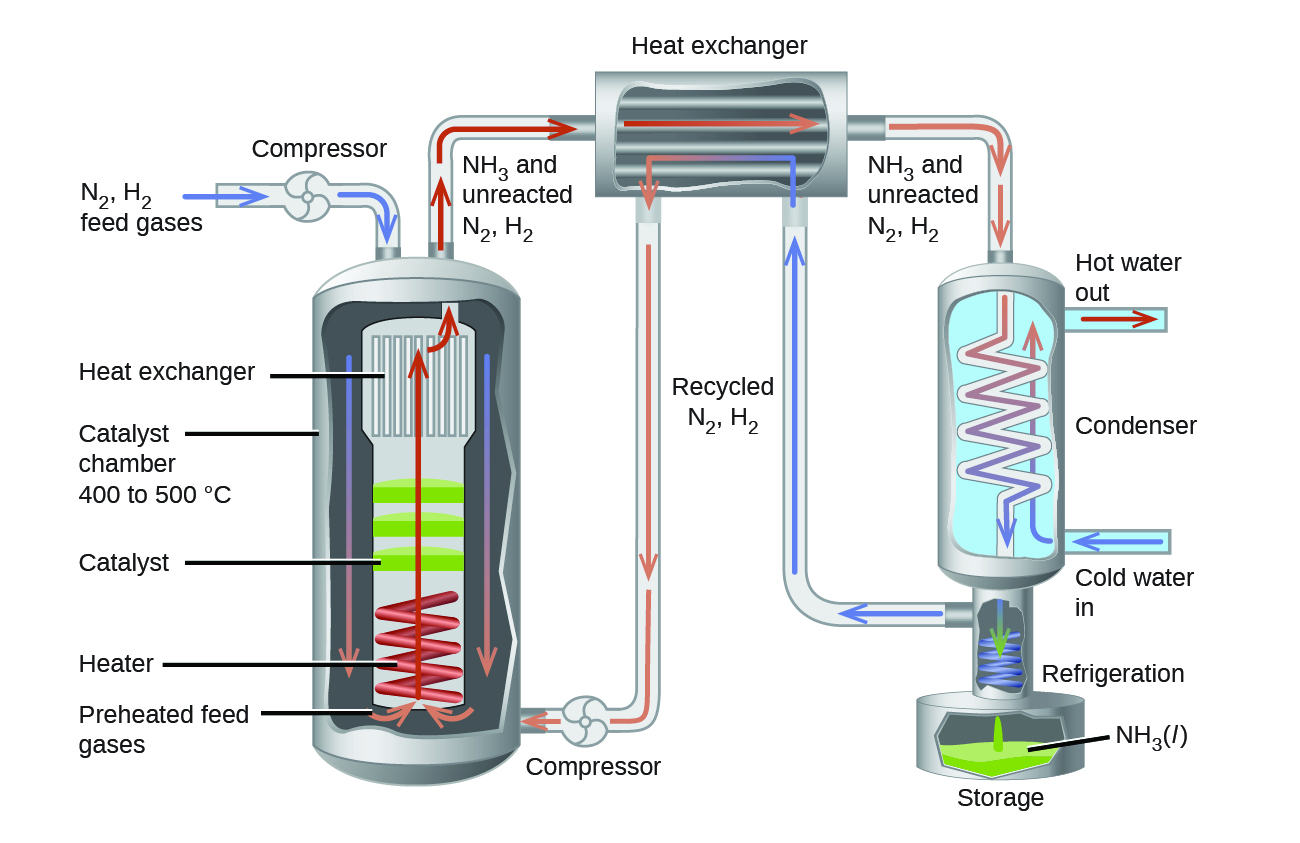### Key Concepts and Summary

Systems at equilibrium can be disturbed by changes to temperature, concentration, and, in some cases, volume and pressure. The system’s response to these disturbances is described by Le Châtelier’s principle: An equilibrium system subjected to a disturbance will shift in a way that counters the disturbance and re-establishes equilibrium. A catalyst will increase the rate of both the forward and reverse reactions of a reversible process, increasing the rate at which equilibrium is reached but not altering the equilibrium mixture’s composition (K does not change).

### Chemistry End of Chapter Exercises

The following equation represents a reversible decomposition: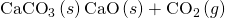Under what conditions will decomposition in a closed container proceed to completion so that no CaCO3 remains?

The amount of CaCO3 must be so small that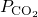is less than KP when the CaCO3 has completely decomposed. In other words, the starting amount of CaCO3 cannot completely generate the fullrequired for equilibrium.

Explain how to recognize the conditions under which changes in volume will affect gas-phase systems at equilibrium.

What property of a reaction can we use to predict the effect of a change in temperature on the value of an equilibrium constant?

The change in enthalpy may be used. If the reaction is exothermic, the heat produced can be thought of as a product. If the reaction is endothermic the heat added can be thought of as a reactant. Additional heat would shift an exothermic reaction back to the reactants but would shift an endothermic reaction to the products. Cooling an exothermic reaction causes the reaction to shift toward the product side; cooling an endothermic reaction would cause it to shift to the reactants’ side.

The following reaction occurs when a burner on a gas stove is lit: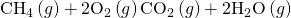Is an equilibrium among CH4, O2, CO2, and H2O established under these conditions? Explain your answer.

No, it is not at equilibrium. Because the system is not confined, products continuously escape from the region of the flame; reactants are also added continuously from the burner and surrounding atmosphere.

A necessary step in the manufacture of sulfuric acid is the formation of sulfur trioxide, SO3, from sulfur dioxide, SO2, and oxygen, O2, shown here. At high temperatures, the rate of formation of SO3 is higher, but the equilibrium amount (concentration or partial pressure) of SO3 is lower than it would be at lower temperatures.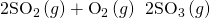(a) Does the equilibrium constant for the reaction increase, decrease, or remain about the same as the temperature increases?

(b) Is the reaction endothermic or exothermic?

Suggest four ways in which the concentration of hydrazine, N2H4, could be increased in an equilibrium described by the following equation: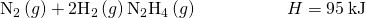Add N2; add H2; decrease the container volume; heat the mixture.

Suggest four ways in which the concentration of PH3 could be increased in an equilibrium described by the following equation: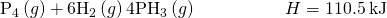How will an increase in temperature affect each of the following equilibria? How will a decrease in the volume of the reaction vessel affect each?

(a)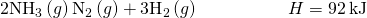(b)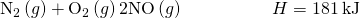(c)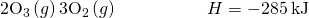(d)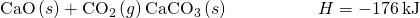(a) T increase = shift right, V decrease = shift left; (b) T increase = shift right, V = no effect; (c) T increase = shift left, V decrease = shift left; (d) T increase = shift left, V decrease = shift right.

How will an increase in temperature affect each of the following equilibria? How will a decrease in the volume of the reaction vessel affect each?

(a)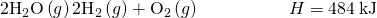(b)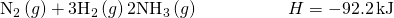(c)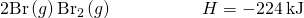(d)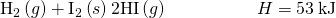Methanol can be prepared from carbon monoxide and hydrogen at high temperature and pressure in the presence of a suitable catalyst.

(a) Write the expression for the equilibrium constant (Kc) for the reversible reaction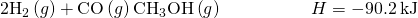(b) What will happen to the concentrations of H2, CO, and CH3OH at equilibrium if more H2 is added?

(c) What will happen to the concentrations of H2, CO, and CH3OH at equilibrium if CO is removed?

(d) What will happen to the concentrations of H2, CO, and CH3OH at equilibrium if CH3OH is added?

(e) What will happen to the concentrations of H2, CO, and CH3OH at equilibrium if the temperature of the system is increased?

(f) What will happen to the concentrations of H2, CO, and CH3OH at equilibrium if more catalyst is added?

(a)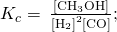(b) [H2] increases, [CO] decreases, [CH3OH] increases; (c), [H2] increases, [CO] decreases, [CH3OH] decreases; (d), [H2] increases, [CO] increases, [CH3OH] increases; (e), [H2] increases, [CO] increases, [CH3OH] decreases; (f), no changes.

Nitrogen and oxygen react at high temperatures.

(a) Write the expression for the equilibrium constant (Kc) for the reversible reaction(b) What will happen to the concentrations of N2, O2, and NO at equilibrium if more O2 is added?

(c) What will happen to the concentrations of N2, O2, and NO at equilibrium if N2 is removed?

(d) What will happen to the concentrations of N2, O2, and NO at equilibrium if NO is added?

(e) What will happen to the concentrations of N2, O2, and NO at equilibrium if the volume of the reaction vessel is decreased?

(f) What will happen to the concentrations of N2, O2, and NO at equilibrium if the temperature of the system is increased?

(g) What will happen to the concentrations of N2, O2, and NO at equilibrium if a catalyst is added?

Water gas, a mixture of H2 and CO, is an important industrial fuel produced by the reaction of steam with red hot coke, essentially pure carbon.

(a) Write the expression for the equilibrium constant for the reversible reaction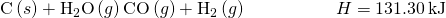(b) What will happen to the concentration of each reactant and product at equilibrium if more C is added?

(c) What will happen to the concentration of each reactant and product at equilibrium if H2O is removed?

(d) What will happen to the concentration of each reactant and product at equilibrium if CO is added?

(e) What will happen to the concentration of each reactant and product at equilibrium if the temperature of the system is increased?

(a)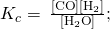(b) [H2O] no change, [CO] no change, [H2] no change; (c) [H2O] decreases, [CO] decreases, [H2] decreases; (d) [H2O] increases, [CO] increases, [H2] decreases; (e) [H2O] decreases, [CO] increases, [H2] increases. In (b), (c), (d), and (e), the mass of carbon will change, but its concentration (activity) will not change.

Pure iron metal can be produced by the reduction of iron(III) oxide with hydrogen gas.

(a) Write the expression for the equilibrium constant (Kc) for the reversible reaction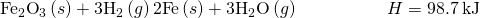(b) What will happen to the concentration of each reactant and product at equilibrium if more Fe is added?

(c) What will happen to the concentration of each reactant and product at equilibrium if H2O is removed?

(d) What will happen to the concentration of each reactant and product at equilibrium if H2 is added?

(e) What will happen to the concentration of each reactant and product at equilibrium if the volume of the reaction vessel is decreased?

(f) What will happen to the concentration of each reactant and product at equilibrium if the temperature of the system is increased?

Ammonia is a weak base that reacts with water according to this equation: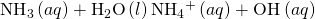Will any of the following increase the percent of ammonia that is converted to the ammonium ion in water?

(a) Addition of NaOH

(b) Addition of HCl

(c) Addition of NH4Cl

Only (b)

Acetic acid is a weak acid that reacts with water according to this equation: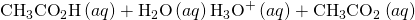Will any of the following increase the percent of acetic acid that reacts and produces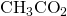ion?

(a) Addition of HCl

(b) Addition of NaOH

(c) Addition of NaCH3CO2

Suggest two ways in which the equilibrium concentration of Ag+ can be reduced in a solution of Na+, Cl, Ag+, and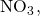in contact with solid AgCl.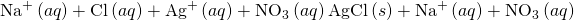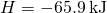Add NaCl or some other salt that produces Cl to the solution. Cooling the solution forces the equilibrium to the right, precipitating more AgCl(s).

How can the pressure of water vapor be increased in the following equilibrium?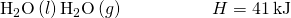A solution is saturated with silver sulfate and contains excess solid silver sulfate: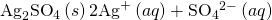A small amount of solid silver sulfate containing a radioactive isotope of silver is added to this solution. Within a few minutes, a portion of the solution phase is sampled and tests positive for radioactive Ag+ ions. Explain this observation.

(a)

The amino acid alanine has two isomers, α-alanine and β-alanine. When equal masses of these two compounds are dissolved in equal amounts of a solvent, the solution of α-alanine freezes at the lowest temperature. Which form, α-alanine or β-alanine, has the larger equilibrium constant for ionization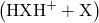?

### Glossary

Le Châtelier’s principle
an equilibrium subjected to stress will shift in a way to counter the stress and re-establish equilibrium

## LicenseChemistry 2e by OSCRiceUniversity is licensed under a Creative Commons Attribution 4.0 International License, except where otherwise noted.